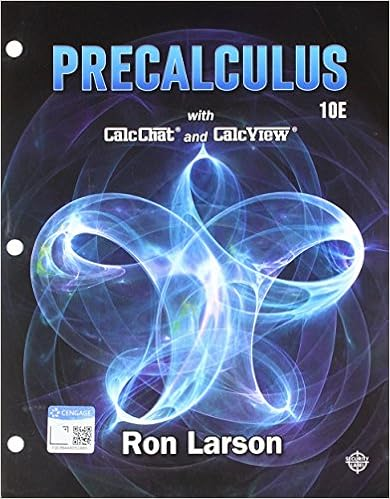Question: Factor by grouping 4z 2 + 12z - az –...

• Homework Help
• 2
• 67% (6) 4 out of 6 people found this document helpful

This preview shows page 1 - 2 out of 2 pages.

We have textbook solutions for you!
The document you are viewing contains questions related to this textbook.The document you are viewing contains questions related to this textbook.
Chapter 2 / Exercise 1
Precalculus
Hostetler/LarsonExpert Verified
MATH 114N Week 1 Section 13.2 Homework 1. Question: Factor by grouping. 4z 2 + 12z - az – 3a 4z 2 + 12z - az – 3a = (z + 3)(4z - a) 2. Question: Which is the correct factored form of x 2 – 13x + 42? 3. Question: What polynomial can be factored as (a + 9)(a + 3)? 4. Question: List all the pairs of integers with a product of 48.
We have textbook solutions for you!
The document you are viewing contains questions related to this textbook.The document you are viewing contains questions related to this textbook.
Chapter 2 / Exercise 1
Precalculus
Hostetler/LarsonExpert Verified
•••